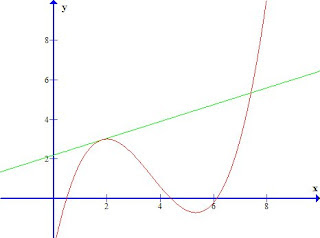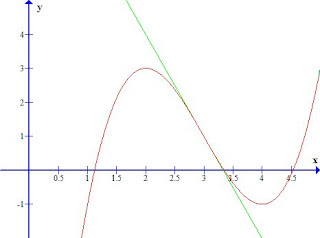img.latex_eq { padding: 0; margin: 0; border: 0; }

## Saturday, 5 January 2008

### Lightning-Run Calculus: The Derivative

Bad news, oh ye who tremble in the presence of mathematical symbols! I had this cool idea for a maths post. And then I realised it involved multivariate calculus. And I didn't want to leave anybody out, so I thought I'd make a lightning-run post so that those who don't know any calculus and want to sort of have an idea of what I'm talking about should at least be able to get some of the ideas into your head.

I know that nobody who knows nothing of calculus is going to be able to pick up all the ideas presented in this post, all at once. I also know I'm not quite telling you everything you need to know to understand my post above. However, I figure that if you thought you vaguely understood something in the post I'm about to make after this one, you might be able to check in this post to see if you were thinking along the right lines.

At the bottom line, I just like having this stuff here for completeness.

I'm not quite sure if I can help anyone who is too afraid of algebra to understand the following:

Let m be the number of biscuits Mary ate. Let n be the number of biscuits Nathan ate. Between then, Mary and Nathan ate 12 biscuits in total. Therefore, m + n = 12.

You don't have to be able to figure out whan m and n are. In fact, in case you haven't noticed, you can't, because I haven't given you enough information. But I'm going to be working on the level of "getting the basic idea" rather than actually being able to calculate things, and I'm going to assume that you get the basic idea of how letters substitute for numbers in algebra. I'm also going to assume that you sort of get how we make a graph of a function like, say,. I mean, you might not be able to draw it off the top of your head, but I'm hoping you'd have a rough idea of what sort of a picture we're talking about, and you'd know that points on the curvy line drawn by the graph correspond to values of y, as calculated by that function, for given values of x.

Ready?

Derivatives

The derivative of a function at a point is the slope of the tangent line at that point. Here's a nice picture:Picture created with the help of a piece of open-source software available here.

The red line is the function; the green line is the tangent line for the point x=1.8 . It just touches the graph of the function at that point without actually crossing it. As a result, at that point x=1.8, the function and the tangent line seem to be travelling in the same direction momentarily. By contrast, further to the right at x = roughly 7.2ish, the lines touch and cross each other. The green line is not a tangent to the red line at that point.

For the sake of accuracy, I'm forced to inform you that the tangent line at a particular point can cross the function at that very point. However, when that happens, it looks nothing like the right hand touching point above where the two lines cross at an angle. It looks like this:The basic idea is that the tangent line shows you what the function would do if it stopped bending at that point and just carried on in a straight line in the direction it was pointing at the time. It tells you what direction the function is going at that particular point. There's a perfectly specific and accurate way of defining it, but I'm not going to go there. That's not because it's all that terribly hard; I just don't want to overload you with too many concepts. If you want to understand more fully, find a calculus textbook and/or ask me enough questions in the comments section to convince me you're enthusiastic.

The slope of the tangent line is a number that measures how steep it is. A vertical line would have infinite slope; a horizontal line would have zero slope. If the slope of the tangent line is a big number (i.e. if the derivative is large) then both the function and its tangent line are travelling upwards very fast at that point. If the slope of the tangent line (= the derivative) is close to zero, then the function is nearly horizontal at that point. If it's a negative number, the function is travelling downwards. Got it? Don't worry, I won't be testing you later. You should still go through and check that you understand the idea here, though.

We write the derivative like this:By which we mean:
"The derivative of y with respect to x"
or,
"What we get when we differentiate y with respect to x"
(Differentiation is the process that takes a function and gives you its derivative)
or, equivalently,
"How fast y changes as we increase x"

There is an explanation for this seemingly strange notation, but it would require me to go into the subject in greater depth than I intended for this post.

Why do we care about this? Well, any time we want to talk mathematically about how something is changing, derivatives are relevant. For example, if the exchange rate is rising fast, then the tangent line to the exchange rate will have a big slope. We can use the derivative of the exchange rate as a way of factoring changes in the exchange rate into an economic model. If we want to talk about how fast the position of an object is changing -- that is, if we want to talk about an object's speed -- then we need to look at the derivative of a graph of the object's position. If we want to talk about how fast the speed is changing -- that is, if we want to talk about acceleration -- we look at the derivative of the speed. That is, the derivative of the derivative of the position.

It's no coincidence that physics took off after Newton discovered calculus. There was a direct causal relationship (more than one causal relationship in more than one direction, perhaps). This is very useful maths. It's about as useful as mathematics could possibly get.

Partial derivatives

Sometimes, in multivariate calculus, you have a function that depends on more than one variable. For example, you might want a function that can tell you how much petrol a car will use based on how fast it is going and how much weight it is carrying. Or you might want to make a graph of altitude based on latitude and longitude. If you want some three-dimensional pictures of functions (graphed along the vertical z axis) that depend on two variables (graphed on the x and y axes), you can go here and type in some expressions in x and y into the box on the upper right that has 'z = ' to the left of it. Try looking at, you know, z = x^2+y^2+3*x^3 or something (That's computer keyboard speak for, in case you didn't know -- you use the * instead of an x for multiplication. I know I'm being really pedantic here but I'm trying to be cautious).

There are several ways of finding a derivative in this situation. The simplest way, or at least the one you learn first, is to introduce the idea of partial derivatives. First you ignore the fact that you can travel in the y direction and just try traveling in the x direction. The slope in that direction gives you the partial derivative of z with respect to x:We use a curly 'd' so we know there are other things that could also cause the z value to vary, not just x.

You can also find the slope in the y direction, pretending x doesn't change and just seeing how fast z changes as you increase y. The two partial derivatives together can be used to give you general information about the slope of the function at that point in any given direction.

In the specific example of the car, we could see how the amount of petrol being used changes as the speed is varied, for a given weight carried, by taking the partial derivative of the amount of petrol being used with respect to changes in speed. Or if we wanted to know how fast the amount of petrol being used would change as we changed the weight carried, for a given speed, we'd take the partial derivative of petrol with respect to weight.

The Chain Rule

One last thing. The chain rule. In the ordinary case where you just have a function y that depends on a single variable x, it goes like this. Suppose x is also a function of some variable t. So for any given value of t, we can put that into a function to work out the value of x, and then we can put that x value into a different function to give us y.

t --> x --> y

The chain rule exists to deal with the following question. Suppose we know how fast x changes as t changes, and suppose we know how fast y changes as x changes. How fast does y change as t changes? The chain rule can tell us.

In the case where you have a function depending on two variables, it gets more complicated. You can have z depending on x and y, which both depend on t -- so for any given t value there will be x and y values which will then give you a specific value of z for that t value -- so there's a 'chain rule' to work out the derivative of z with respect to t based on the partial derivative of z with respect to x and y, and the derivatives of x and y with respect to t. Or it's not uncommon to have x and y both depending on the same pair of variables u and v. In that case, you might want to work out the partial derivative of z with respect to u or to v. The chain rule is easily adapted to let us use the partial derivatives of x and y with respect to u or v to tell us.

I haven't told you how to actually calculate any of this. (In keeping with that, I haven't told you in this post what the chain rule actually is!) Largely, I haven't told you that part of things because becoming proficient at the calculational side takes practice. This means you won't be able to understand the calculations in my post above. You might get an inkling of the concepts, though.

#### 3 comments:Anonymous said...

I must confess, the only part of this that I understand at all is the chain rule, which I recognize as the hypothetical syllogism of symbolic logic.

Lynet said...

Oh, well. I tried. It was quite fun to write, in any case.

jklarson said...

I am starting my own blog about derivatives in calculus!! It's for my education technology class, so I enjoyed this page!!!